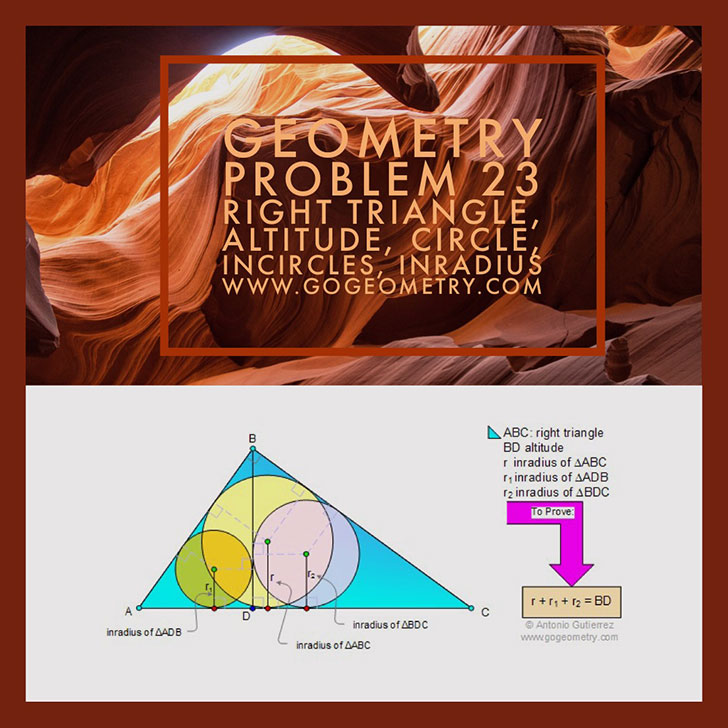# Geometry Problem 23 with Solution: Right Triangle, Altitude, Circle, Incircles, Inradius

 < PREVIOUS PROBLEM  |  NEXT PROBLEM > In triangle ABC, angle B is a right angle and BD is the altitude. Let r, r1, and r2 be the inradii of triangles ABC, ADB, and BDC respectively. Prove that r + r1 + r2 = BD.## Poster and Typography of Problem 23Home | Search | Geometry | Problems | All Problems | Open Problems | Visual Index | 10 Problems | Problems Art Gallery |  Art | 21-30 | Circles | Right Triangle | Incircle | Circle Tangent Line | Right triangle, Altitude and Incircle | by Antonio Gutierrez Last updated: Sep 19, 2014 Post or view a solution to the problem 23